9.1 广义可加模型¶

• $g(\mu)=\mu$ 是等值链接，用于高斯响应数据的线性和可加模型
• 如上面所示的对二项分布概率建模的 $g(\mu)=\logit(\mu)$，或者 $g(\mu)=\probit(\mu)$ （probit 链接函数）．probit 函数是高斯分布函数的反函数：$\probit(\mu)=\Phi^{-1}(\mu)$
• $g(\mu)=\log(\mu)$ 应用于处理泊松计数数据的对数线性或者对数加性模型．

• $g(\mu)=X^T\beta+\alpha_k+f(Z)$，这是一个半参模型，其中 $X$ 是需要线性建模的预测变量的向量，$\alpha_k$ 是定性输入变量 $V$ 的第 $k$ 个层次的影响，而且非参数地建模预测变量 $Z$ 的影响．
• $g(\mu)=f(X)+g_k(Z)$，同样 $k$ 表示定性输入 $V$ 的层次，因此这构造出一个关于 $V$ 和 $Z$ 影响的交叉项 $g(V,Z)=g_k(Z)$
• $g(\mu)=f(X)+g(Z,W)$，其中 $g$ 是关于两个特征的非参数函数．

拟合可加模型¶

weiya 注: Recall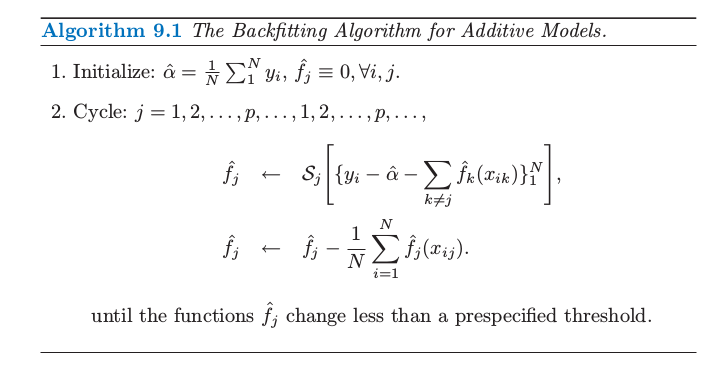weiya 注：Ex. 9.1

• 其它的单变量回归光滑器，比如局部多项式回归和核方法；
• 线性回归算子，可以得到多项式拟合，分段常值拟合，参数化样条拟合，序列和傅里叶拟合；
• 更加复杂的算子，比如对于二阶或者高阶交互项或者对于季节影响的周期光滑器．

weiya 注：Ex. 9.2

例子：可加逻辑斯蒂回归¶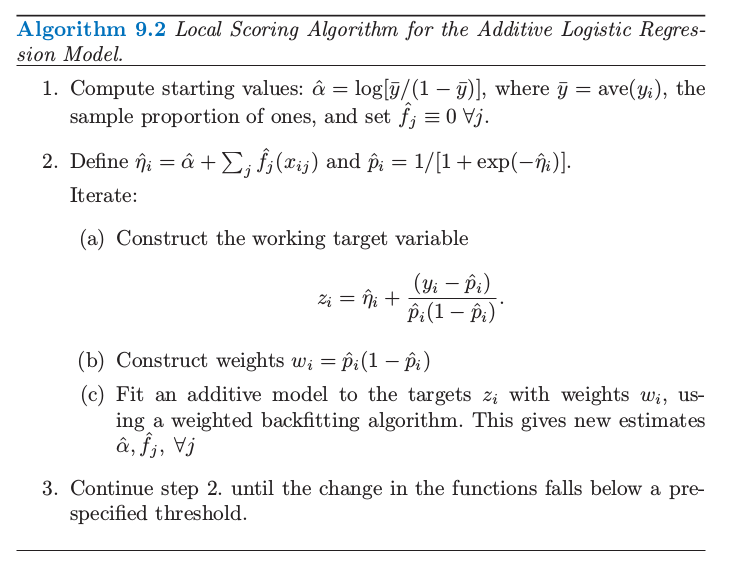http://www.stat.auckland.ac.nz/∼yee

例子：预测垃圾邮件¶

• 6 个定量预测变量——邮件中匹配给定字符的字符比例．字符为 ch;ch(ch[ch!ch$，和 ch# • 未间断的大写字符序列的平均长度：CAPAVE • 未间断的大写字符序列的最长长度：CAPMAX • 未间断的大写字符序列的总长度：CAPTOT 我们将 spam 编码成 1，email 编码成 0．随机选取大小为 1536 的测试集，剩下的 3065 个测试集作为测试集．一个广义加性模型通过对每个预测变量用四个名义自由度的三次光滑样条．这意味着对于每个预测变量$X_j$，选择光滑样条的参数$\lambda_j$使得$\mathrm {trace}[\mathbf S_j(\lambda_j)]-1=4$，其中$\mathbf S_j(\lambda)$是使用观测数据$x_{ij},i=1,\ldots,N$构造出的光滑样条算子矩阵．这是在这样一个复杂模型中确定光滑数量的方便方式． 大多数的 spam 预测变量服从一个非常长的长尾分布．在拟合 GAM 模型之前，对每个变量进行对数变换（实际上是$\log(x+0.1)$），但是在图 9.1 画出的是关于原始变量的函数．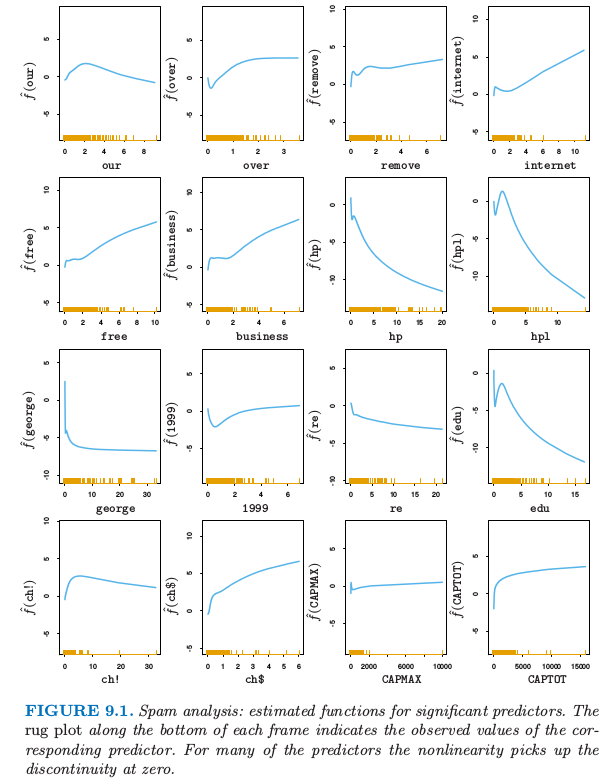图 9.1. 垃圾邮件分析：对于显著性预测变量的估计函数．在每张图的横轴的须状图 (rug plot) 表示对应的预测变量．对于许多预测变量非线性是在 0 处的不连续性导致的． 表 9.1 显示了测试误差率；总体误差率为$5.3\%$．为了对比，线性逻辑斯蒂回归有$7.6\%$的测试误差．表 9.2 显示了在可加性模型中显著性强的预测变量．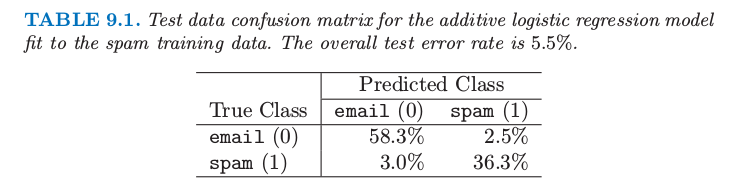表 9.1. 对垃圾训练数据的可加逻辑斯蒂回归模型拟合的混淆矩阵．整体测试误差率为$5.5\%$.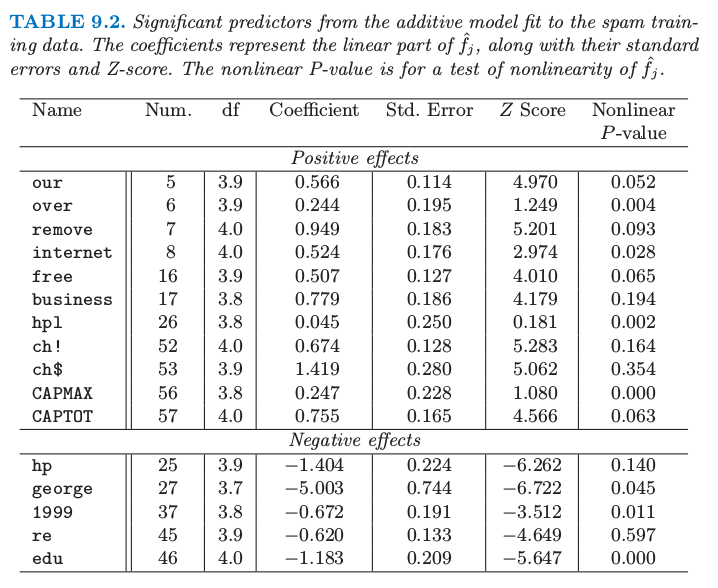表 9.2. 对 spam 训练数据进行可加模型拟合的显著性预测变量．系数和它们的标准误差以及 Z 得分来表示$\hat{f_j}$的线性部分．非线性 p 值是对$\hat{f_j}$非线性的检验． 为了解释的方便，表 9.2 中有每个变量分解成线性组分和剩余非线性组分的贡献．靠前的预测变量与 spam 正相关，而最后的预测变量负相关．线性组分是在预测变量上对拟合曲线的加权最小二乘线性拟合，而非线性部分是剩余部分．估计函数的线性部分是由系数、标准误差和$Z$得分来总结（表示）；后者（指 Z 得分）是系数除以它的标准误差，而且当它超过合适的标准正态分布的分位数认为是显著的．标签为 nonlinear P-value 的列是对估计函数非线性部分的假设检验．然而，注意到，每个预测变量的影响是根据其他变量的整体影响来调整的，而不仅仅是它们的线性部分．表中显示的预测变量通过在$p=0.01$水平下（双边）进行至少一个检验（线性或者非线性）来判断是否显著的． 图 9.1 显示了在表 9.2 中出现的显著预测变量的估计函数．许多非线性影响表明在 0 处的强不连续性．举个例子，当 george 的频率从$0$增长， spam 的概率显著下降，但是此后没有太大改变．这表明或许会用一个表示计数为 0 的指示变量来替换每个频率预测变量，这样回到线性逻辑斯蒂回归．这给出$7.4\%$的测试误差；包括频率的线性影响将测试误差降低到$6.6\%$．看起来在可加模型中的非线性有着额外的预测力量． 将真正的 email 分类成 spam 是很严重的，因为好邮件会被过滤掉而不能到达用户手中．我们可以通过改变损失来平衡类别误差率（见 2.4 节）．如果我们对将真实的类别 0 预测成类别 1 赋予损失$L_{01}$，$L_{10}$为将真实的类别 1 预测成类别 0 的损失，于是如果概率大于$L_{01}/(L_{01}+L_{10})$则估计的贝叶斯准则预测为类别 1．举个例子，如果我们取$L_{01}=10,L_{10}=1$则（真实的）类别 0 和类别 1 误差率变成$0.8\%$和$8.7\%$． 更 ambitiously，我们通过对类别 0 的观测赋予权重$L_{01}$，对类别 1 的观测赋予权重$L_{10}$使得模型更好地对类别 0 中的数据进行拟合．像上面一样，我们接着运用贝叶斯准则来预测．在类别 0 和类别 1 中误差率分别为$1.2\%$和$8.0\%$．我们将在基于树的模型中讨论损失不相等的问题． 拟合加性模型之后，应该检查一下加入交互关系之后是否显著性提高拟合．这可以通过插入一些或全部的显著性输入来“手动”完成，或者通过 MARS 过程来自动完成．（见 9.4 节 这个例子以一种自动的方式来使用加性模型．作为一个数据分析工具，可加模型经常以一种更交互的方式来使用，通过添加或者删除项来确定它们的影响．通过校准关于$df_j$的光滑数量，可以在线性模型 ($df_j=1\$) 和部分线性模型中移动，其中对一些项更加灵活地建模．更多细节见 Hastie and Tibshirani(1990)2

总结¶

1. Buja, A., Hastie, T. and Tibshirani, R. (1989). Linear smoothers and additive models (with discussion), Annals of Statistics 17: 453–555

2. Hastie, T. and Tibshirani, R. (1990). Generalized Additive Models, Chapman and Hall, London.

3. Yee, T. and Wild, C. (1996). Vector generalized additive models, Journal of the Royal Statistical Society, Series B. 58: 481–493.

4. Lin, Y. and Zhang, H. (2006). Component selection and smoothing in smoothing spline analysis of variance models, Annals of Statistics 34: 2272–2297.

5. Ravikumar, P., Liu, H., Lafferty, J. and Wasserman, L. (2008). Spam: Sparse additive models, in J. Platt, D. Koller, Y. Singer and S. Roweis (eds), Advances in Neural Information Processing Systems 20, MIT Press, Cambridge, MA, pp. 1201–1208.